In :
###########################################################################
# An example of an object classification/categorization system
# using a CNN on color images from the dataset CIFAR-10.
# Adapted from the PyTorch Tutorials, "Training a Classifier"
###########################################################################

import torch, torchvision
import torchvision.transforms as transforms

# The output of torchvision datasets are PILImage images of range [0, 1].
# Transform them to Tensors of normalized range [-1, 1]
transform = transforms.Compose(
[transforms.ToTensor(),
transforms.Normalize((0.5, 0.5, 0.5), (0.5, 0.5, 0.5))])
# Classes of images
classes = ('plane', 'car', 'bird', 'cat', 'deer', 'dog', 'frog', 'horse', 'ship', 'truck')
# Read in the training & testing data
iterBatchSize = 5

Files already downloaded and verified

In :
# What does the training data look like?
import matplotlib.pyplot as plt
import numpy as np
# Functions to show an image
def imshow(img):
img = img / 2 + 0.5  # unnormalize
plt.imshow( np.transpose(img.numpy(), (1, 2, 0)) )
# Let's look at some example training images
dataiter = iter(trainloader) # For training images
testdataiter = iter(testloader) # For testing images (used later)
images, labels = dataiter.next() # Ask the iterator for some images
# Show images and labels
imshow(torchvision.utils.make_grid(images))
plt.show()
print(', '.join('%s' % classes[labels[j]] for j in range(iterBatchSize)))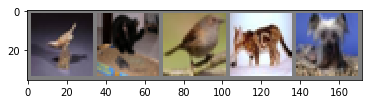bird, cat, bird, cat, dog

In :
# Now we can define our model

import torch.nn as nn
import torch.nn.functional as F

# Define a small CNN that processes 3-channel images
class Net(nn.Module):
# Initialize our layers, i.e. the set of trainable parameters
def __init__(self):
super(Net, self).__init__()
# A convolutional layer
# The number of input channels is 3 this time (rgb)
self.conv1 = nn.Conv2d(3, 6, 5)
# A max pooling layer (will be reused for each conv layer)
self.pool = nn.MaxPool2d(2, 2)
# Another convolutional layer
self.conv2 = nn.Conv2d(6, 16, 5)
# Three sets of fully connected (linear) layers
self.fc1 = nn.Linear(16 * 5 * 5, 120)
self.fc2 = nn.Linear(120, 84)
self.fc3 = nn.Linear(84, 10)

def forward(self, x):
# For each conv layer: conv -> relu -> pooling
x = self.pool(F.relu(self.conv1(x)))
x = self.pool(F.relu(self.conv2(x)))
# Reshape from higher dimensional tensor to a vector for the FC layers
x = x.view(-1, 16 * 5 * 5)
# Pass through fully connected layers
x = F.relu(self.fc1(x))
x = F.relu(self.fc2(x))
x = self.fc3(x)
return x

model_saved_name = 'cifar10-net.model'
net = Net()

In :
# Look at the weights and network structure
print(net.state_dict().keys())
print("Conv1:",net.conv1.weight.size())
print("Conv2:",net.conv2.weight.size())
print(net.conv1.weight[3,:,:,:])
print(net.conv2.weight.size())
print(net.conv2.weight[3,:,:,:])

odict_keys(['conv1.weight', 'conv1.bias', 'conv2.weight', 'conv2.bias', 'fc1.weight', 'fc1.bias', 'fc2.weight', 'fc2.bias', 'fc3.weight', 'fc3.bias'])
Conv1: torch.Size([6, 3, 5, 5])
Conv2: torch.Size([16, 6, 5, 5])
Variable containing:
(0 ,.,.) =
0.0369  0.0161  0.0546 -0.0537  0.0306
-0.0539  0.0948  0.1071  0.0570 -0.1076
-0.1090 -0.0591  0.0414 -0.0718 -0.0102
-0.0295  0.0846 -0.0686  0.0246 -0.0243
-0.1011 -0.0031 -0.0068 -0.0017 -0.0477

(1 ,.,.) =
-0.0259 -0.1139  0.0673  0.0470 -0.1075
-0.1117 -0.0230  0.0268 -0.0366  0.0251
-0.0288  0.0239  0.0819 -0.0938  0.0665
-0.0659 -0.0185  0.0842  0.0779 -0.0375
0.1091 -0.1136  0.0321  0.0786 -0.0656

(2 ,.,.) =
0.0633 -0.0088  0.0623 -0.0236  0.0206
0.0070  0.0551  0.0533 -0.0621 -0.0118
-0.0579 -0.1056  0.0271 -0.0683 -0.0894
0.0844 -0.0459 -0.0991  0.0873 -0.0708
0.0835 -0.0460 -0.0236  0.0308  0.0895
[torch.FloatTensor of size 3x5x5]

torch.Size([16, 6, 5, 5])
Variable containing:
(0 ,.,.) =
1.00000e-02 *
7.0983 -4.5930  4.2059 -2.4674  4.7207
-6.0061 -1.0516 -2.2923 -5.9053  1.0691
-0.0811 -0.2345 -6.7966 -6.8837 -2.9125
5.4543  2.1295 -0.1932 -5.5605  0.8616
-7.8767 -6.5717 -3.1128 -5.8034  5.2758

(1 ,.,.) =
1.00000e-02 *
-1.6839  3.8318  6.9414  7.7306  5.8249
5.8795  7.4263  2.7641 -3.3969 -0.3972
1.1386 -1.9550 -6.2595  0.2811  3.9524
-4.6918 -0.8927 -1.9259 -2.2477 -0.9632
4.8702 -8.0973 -7.0787 -3.8013  6.7551

(2 ,.,.) =
1.00000e-02 *
7.3275  2.0496 -7.8671  2.8689  1.5039
-1.4734  3.9806 -6.2667 -7.2686 -3.4736
-6.6012  4.3638 -7.9813  3.0558  5.5389
1.0744  7.4173  6.3720  4.2709 -6.1855
2.9651  0.7073  2.5341  5.1997  5.5284

(3 ,.,.) =
1.00000e-02 *
2.4051 -6.7769 -4.7964  0.2031 -6.2922
0.9757  7.1526  3.0293 -3.3110  7.5623
2.3571  3.3864 -6.1725  3.1885 -1.2919
-1.2573  7.8206  7.6680  2.3228  2.8274
-2.3443 -7.8567  4.0741 -7.9160  6.0846

(4 ,.,.) =
1.00000e-02 *
-7.4472  6.4949 -7.4266 -0.2276  7.2885
5.4237 -0.6710 -7.2233  3.7984  1.5126
-4.0209 -3.4991  2.2636 -3.5541  0.0845
2.8845 -0.2394 -7.5840  5.7282  3.2346
-5.3066 -0.7957  5.1779  4.0787  5.2748

(5 ,.,.) =
1.00000e-02 *
-4.5250 -6.2591  1.1848 -0.9412  3.1640
6.6999  0.5121 -2.9684 -3.6494 -2.5125
-8.1094  1.4826 -0.0445 -7.8288 -6.0221
-7.8874  7.3397  1.2175 -7.1306  1.8217
-1.2590  3.6021 -8.0988 -0.9169  1.7901
[torch.FloatTensor of size 6x5x5]


In :
from torchvision import utils
# Define a method to help visualize the convolutional kernels
# From: https://github.com/pedrodiamel/nettutorial/blob/master/pytorch/pytorch_visualization.ipynb
def vistensor(tensor, ch=0, allkernels=False, ncol=8, padding=1):
''' @ch: visualization channel
@allkernels: visualization all tensores
'''
n,c,w,h = tensor.shape
if allkernels: tensor = tensor.view(n*c,-1,w,h )
elif c != 3: tensor = tensor[:,ch,:,:].unsqueeze(dim=1)
rows = np.min( (tensor.shape//ncol + 1, 64 )  )
plt.figure( figsize=(ncol,rows) )
plt.imshow(grid.numpy().transpose((1, 2, 0)))
plt.show()

# Visualize the parameters of tensors before training (just noise)
vistensor(net.conv1.weight.data) # Can view the first conv layer's kernels as being RGB
# Or treat each of the 6 units as having 3 greyscale filters
vistensor(net.conv1.weight.data, allkernels=True, ncol=3)
# The second layer's filters
vistensor(net.conv2.weight.data, allkernels=True, ncol=16) #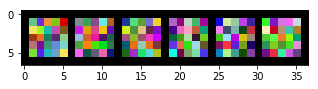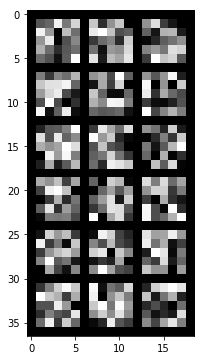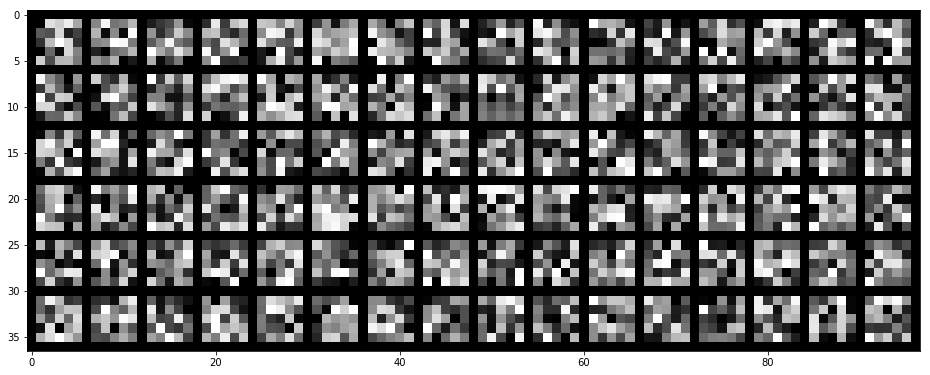In :
import torch.optim as optim
criterion = nn.CrossEntropyLoss()
optimizer = optim.SGD(net.parameters(), lr=0.001, momentum=0.9)

In :
import os
if os.path.exists(model_saved_name):
# Otherwise train it from scratch
else:
for epoch in range(3):  # loop over the dataset multiple times
running_loss = 0.0
for i, data in enumerate(trainloader, 0):
# get the inputs
inputs, labels = data
# wrap them in Variable
inputs, labels = Variable(inputs), Variable(labels)
# forward + backward + optimize
outputs = net(inputs)
loss = criterion(outputs, labels)
loss.backward()
optimizer.step()
# print statistics
running_loss += loss.data
if i % 2000 == 1999:    # print every 2000 mini-batches
print('[%d, %5d] loss: %.3f' % (epoch + 1, i + 1, running_loss / 2000))
running_loss = 0.0
print('Finished Training')

Loaded model

In :
# Save it if a model file is not already present
if not os.path.exists(model_saved_name):
torch.save(net.state_dict(), model_saved_name)

In :
# Let's see what the network learned on some example images
for _ in range(3):
images, labels = testdataiter.next()
# print images
imshow(torchvision.utils.make_grid(images))
print('GroundTruth: ', ' '.join('%5s' % classes[labels[j]] for j in range(iterBatchSize)))
# Output predicted by our net
outputs = net(Variable(images))
# Predicted class = index of max probability (treating output as probability)
_, predicted = torch.max(outputs.data, 1)
print('Predicted:   ', ' '.join('%5s' % classes[predicted[j]] for j in range(iterBatchSize)))
plt.show()

GroundTruth:    cat  ship  ship plane  frog
Predicted:      cat  ship  ship plane  deer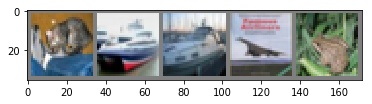GroundTruth:   frog   car  frog   cat   car
Predicted:     frog   dog  frog   cat   car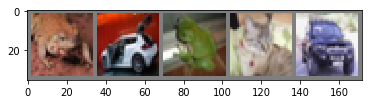GroundTruth:  plane truck   dog horse truck
Predicted:    plane truck horse horse truck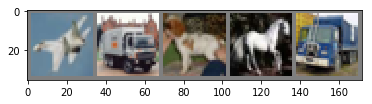In :
# Overall accuracy on the test set
correct, total = 0, 0
images, labels = data
outputs = net(Variable(images))
_, predicted = torch.max(outputs.data, 1)
total += labels.size(0)
correct += (predicted == labels).sum()
print('Accuracy on test set: %d %%' % (100 * correct / total))

Accuracy on test set: 55 %

In :
# What are the accuracies per class?
nClasses = 10
class_correct = list(0. for i in range(10))
class_total = list(0. for i in range(10))
images, labels = data
outputs = net(Variable(images))
_, predicted = torch.max(outputs.data, 1)
c = (predicted == labels).squeeze()
for i in range(iterBatchSize):
label = labels[i]
class_correct[label] += c[i]
class_total[label] += 1
for i in range(nClasses):
print('Accuracy of %5s : %2d %%' % (
classes[i], 100 * class_correct[i] / class_total[i]))

Accuracy of plane : 46 %
Accuracy of   car : 55 %
Accuracy of  bird : 37 %
Accuracy of   cat : 46 %
Accuracy of  deer : 42 %
Accuracy of   dog : 36 %
Accuracy of  frog : 65 %
Accuracy of horse : 81 %
Accuracy of  ship : 77 %
Accuracy of truck : 67 %

In :
# Compute the confusion matrix
from torchnet import meter
confusion_matrix = meter.ConfusionMeter(nClasses)
input, label = data
val_input = Variable(input, volatile=True) #.cuda()
val_label = Variable(label.type(torch.LongTensor), volatile=True) #.cuda()
score = net(val_input)
# Display the confusion matrix
# Perhaps there are some patterns in which ones the classifier gets wrong
a = confusion_matrix.conf
fig = plt.figure()
plt.imshow(a, cmap='hot', interpolation='nearest', aspect='auto')
plt.xticks(list(range(nClasses)))
plt.yticks(list(range(nClasses)))
ax.set_yticklabels(classes)
ax.set_xticklabels(classes)
plt.show() # Rows are ground-truth, cols are predictions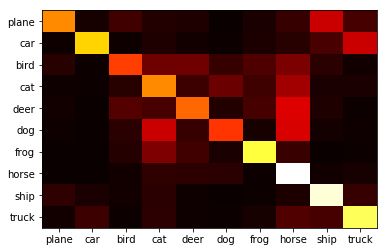In :
# Recall the kernels were gaussian random noise at the start
vistensor(net.conv1.weight.data)
vistensor(net.conv1.weight.data, allkernels=True, ncol=3)
vistensor(net.conv2.weight.data,allkernels=True,ncol=16)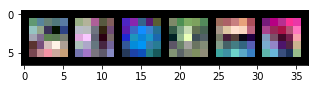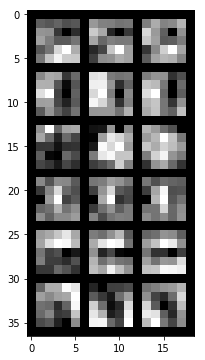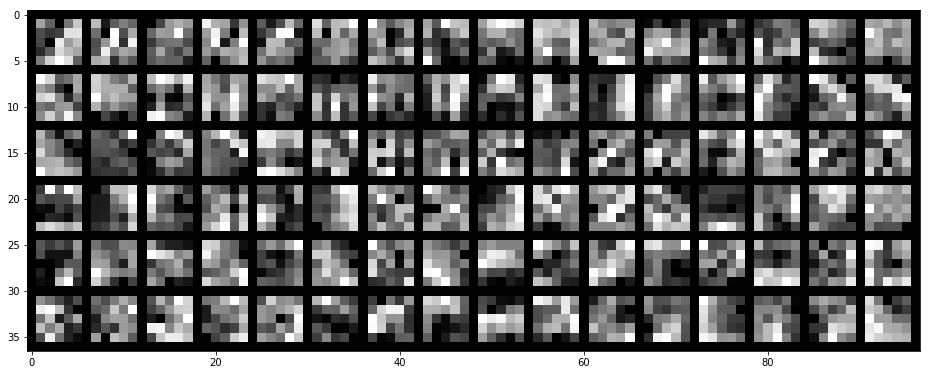In :
# For more visualizations, see: Visualizing and Understanding Convolutional Networks, Zeiler and Fergus, 2014